## MapleSim 2020 is now available!

We’re excited to announce a new version of MapleSim! The MapleSim 2020 family of products lets you build and test models faster than ever, including faster simulations, powerful new tools for machine builders, and expanded modeling capabilities. Improvements include:

• Faster results, with more efficient models, faster simulations, and more powerful design tools.
• Powerful new features for machine builders, with new components, improved visualizations, and automation-focused connectivity tools that make it faster than ever to build and test digital twins.
• Improved modeling capabilities, with an extensive collection of updates to components, libraries, and analysis tools.
• More realistic machine visualizations with an expanded Kinematic Cam Generation App.
• New product: MapleSim Insight, giving machine builders powerful, simulation-based debugging and 3-D visualization capabilities that connect directly to common automation platforms.
• New add-on library:  MapleSim Ropes and Pulleys Library for the easy creation of winch and pulley systems as part of your machine development.

## How to create an interactive Math App in Maple

Maple 2020

Since we are getting many questions on how to create Math apps to add to the Maple Cloud. I wanted to go over the different GUI aspects of how you go about creating a Math App in Maple. The following Document also includes some code examples that are used in the the Math App but doesn't go into them in detail. For more details on the type of coding you do in a Math App see the DocumentTools package help page.

Some of the graphical features of the Math app don't display on Maple Primes so I'd recommend downloading this worksheet from here: HowToMathApp.mw to follow along.How to make a Math App (An example of using the Document Tools).

This Document will provide a beginners guide on one way to make a Math app in Maple.

It will contain some coding examples as well as where to find different options in the user interface.

Step 1 Insert a Table

 • When making a Math App in Maple I often start with a table. You can enter a table by going to Insert > Table...• I often make the table 1 x 2 to start with as this gives an area for input and an area for the output (such as plots).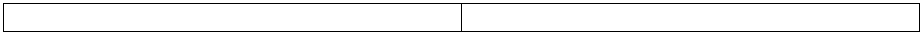Add a plot component to one of the cells of the table

 • From the Components  Palette you can add a Plot Component . Add it to the table by clicking and dragging it over.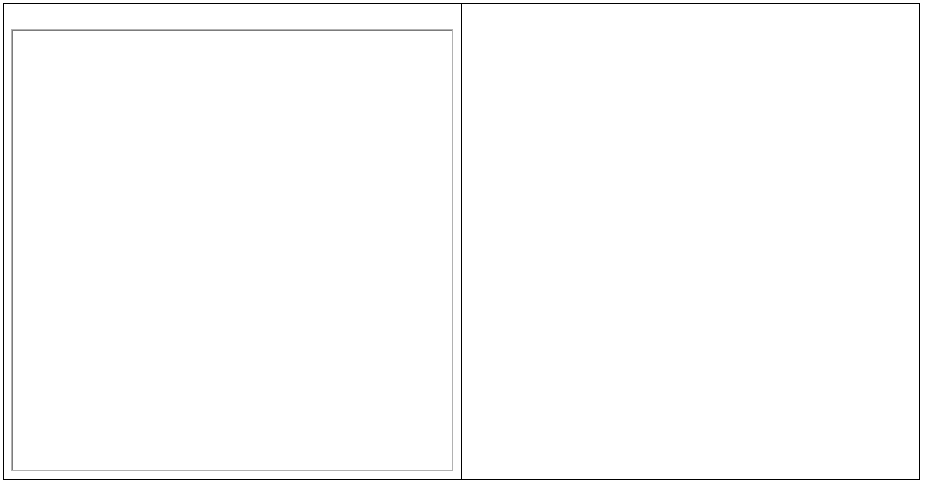Add another table inside the other cell

 • In the other cell of the table I'll add another table to organize my use of buttons, sliders, and other components.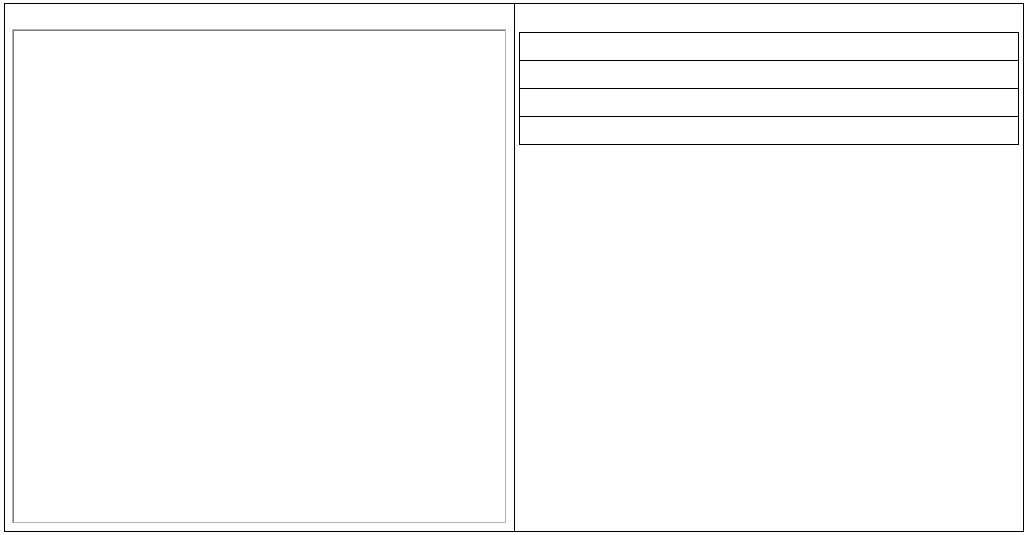Add some components to the new table

 • From the Components Palette I'll add a slider, or dial, or something else for interaction.

 • You may also want a Math region for an area to enter functions and a button to tell Maple to do something with it.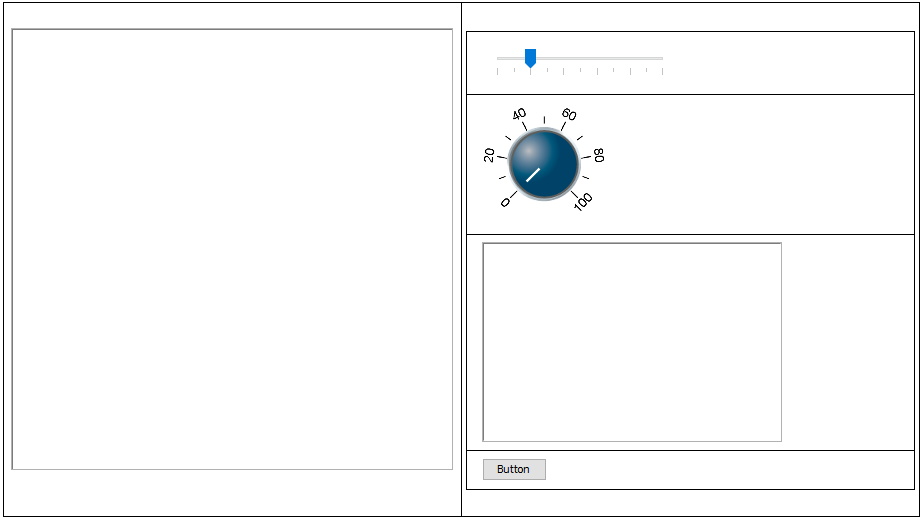Arrange the Components to look nice

 • You can change how the components are placed either by resizing the tables or changing the text orientation of the contents of the cells.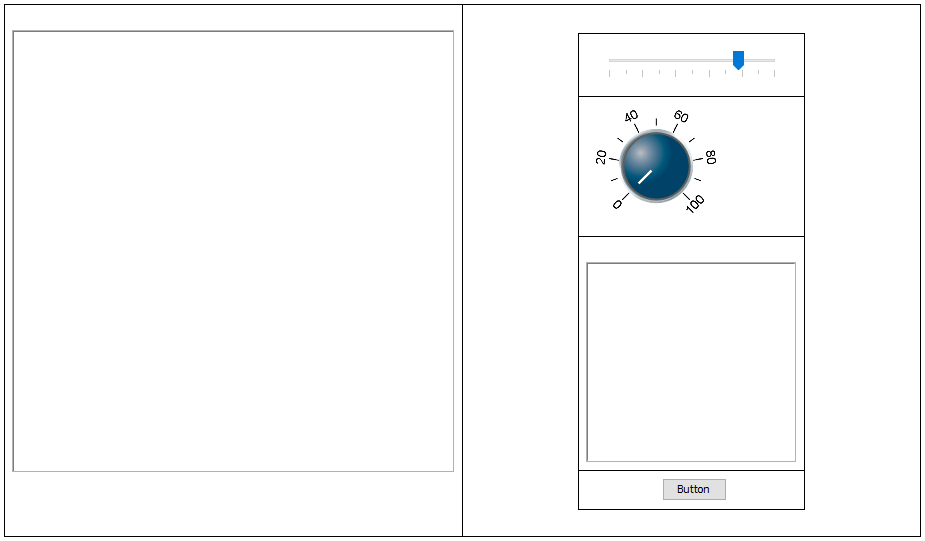Write some code for the interaction of the buttons.

 • Using the DocumentTools  package there are lots of ways you can use the components. I often will start writing my code using a code edit region  as it provides better visualization for syntax. On MaplePrimes these display as collapsed so I will also include code blocks for the code.Let's write something that takes the value of the slider and applies it to the dial

 • Note that the names of the components will change in each section as they are copies of the previous section.

with(DocumentTools):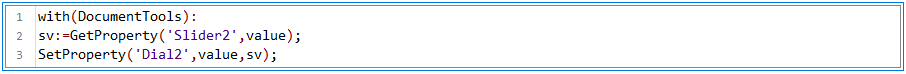```with(DocumentTools):
sv:=GetProperty('Slider2',value);
SetProperty('Dial2',value,sv);```
 • This code will only execute when run using thebutton. Change the value of the slider below then run the code above to see what happens.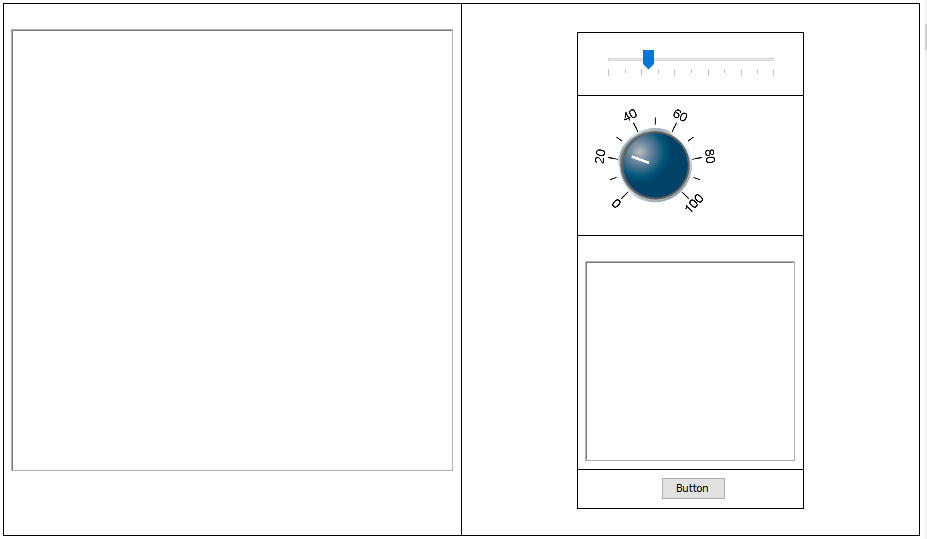Move the code 'inside' the slider

 • Instead of putting the code inside the code edit region where it needs to be executed, we'll next add the code to the value changed code of the slider.

 • Right click the Slider then select "Edit Value Changed Code".• This will open the code editor for the Slider• Enter your code (ensuring you're using the correct name for the slider and dial).

 • Notice that you don't need to use the with(DocumentTools): command as "use DocumentTools in ... end use;" is already filled in for you.

 • Save the code in the Slider and hit thebutton inside it once.
 • Now move the slider.

 • On future uses of the App you won't need to hitas the code will be run on startup.• Let's make this app do something a bit more interesting than change the contents of a dial when a slider moves.

 • The plan in the next few steps is to make this app allow a user to explore parameters changing in a sinusoidal expression.

 • I'm going to add a second Math Component, put the expressioninto both then uncheck the box in the context panel that says "Editable".

 • To make the Math containers fit nicely I'll check the Auto-fit container box and set the Minimum Width Pixels to 200.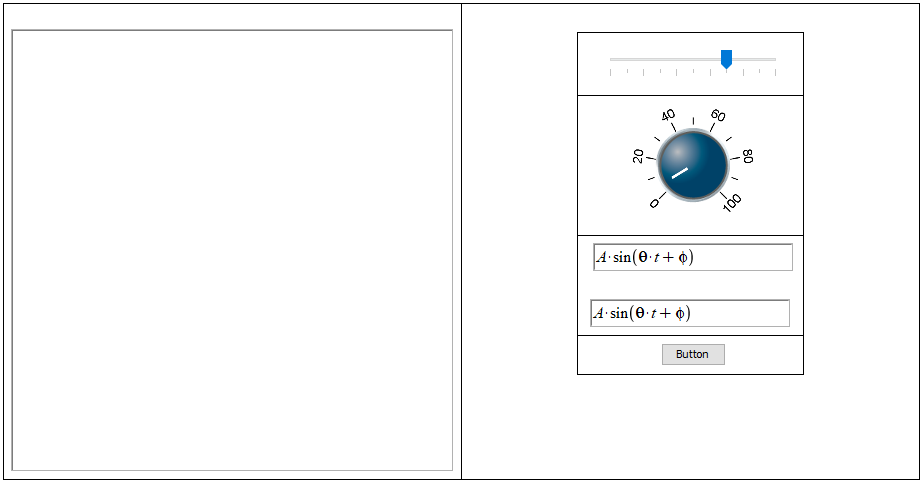Add code to change the value of phi in the second Math Container when the Slider changes Note: Maple uses Radians for trigonometric functions so we should convert the value of phi to Radians. use DocumentTools in   ```use DocumentTools in phi_s:=GetProperty(Slider5,value); expr:= GetProperty(MathContainer6,expression); new_expr:=algsubs(phi=phi_s*Pi/180,expr); SetProperty(MathContainer7,expression,new_expr); end use:```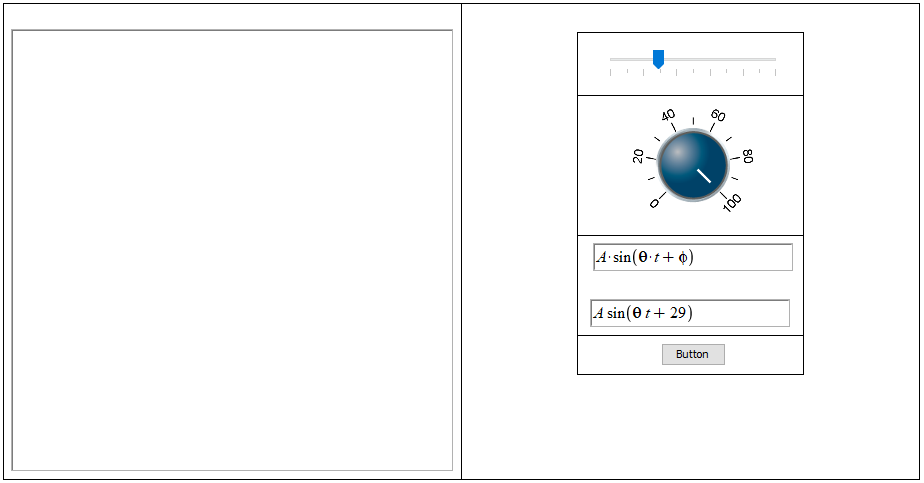Make the Dial go from 0 to 360°

 • Click the Dial and look at the options in the context panel on the right.

 • Update the values in the Dial so that the highest position is 360 and the spacing makes sense for the app.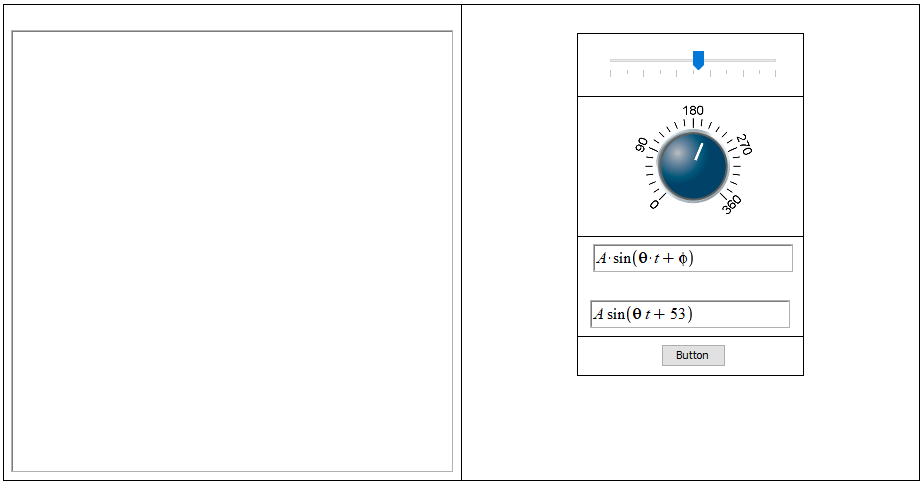Have the Dial update the theta value of the expression

 • Add the following code to the Dial

use DocumentTools in
```use DocumentTools in
theta_d:=GetProperty(Dial7,value);
phi_s:=GetProperty(Slider7,value); #This is added so that phi also has the value updated

expr:= GetProperty(MathContainer10,expression);
new_expr0:=algsubs(theta=theta_d*Pi/180,expr);
new_expr:=algsubs(phi=phi_s*Pi/180,new_expr0);  #This is added so that phi also has the value updated

SetProperty(MathContainer11,expression,new_expr);
end use:
```

 • Update the value in the slider to include the value from the dial

use DocumentTools in

```use DocumentTools in

theta_d:=GetProperty(Dial7,value); #This is added so that theta also has the value updated
phi_s:=GetProperty(Slider7,value);

expr:= GetProperty(MathContainer10,expression);
new_expr0:=algsubs(theta=theta_d*Pi/180,expr); #This is added so that theta also has the value updated
new_expr:=algsubs(phi=phi_s*Pi/180,new_expr0);

SetProperty(MathContainer11,expression,new_expr);

end use:```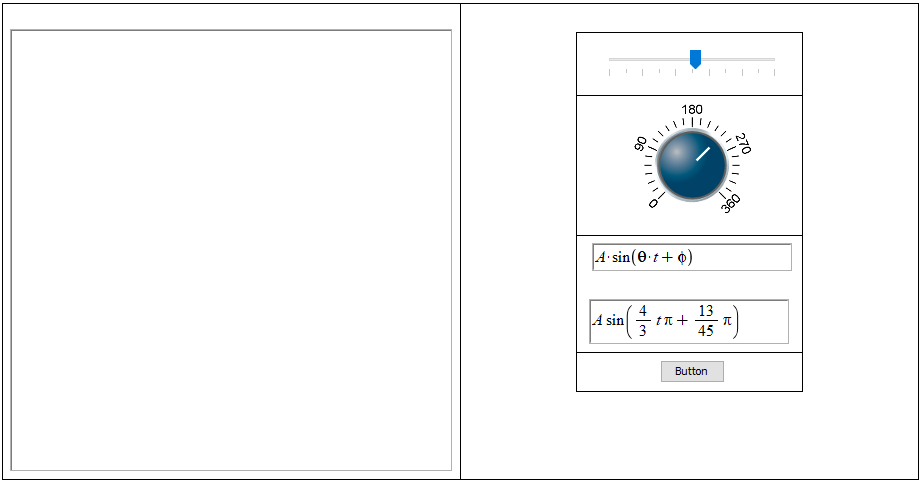Notice that the code in the Dial and Slider are the same

 • Since the code in the Dial and Slider are the same it makes sense to put the code into a procedure that can be called from multiple places.

Note: The changes in the code such as local and the single quotes are not needed but make the code easier to read and less likely to run into errors if edited in the future (for example if you create a variable called dial8 it won't interfere now that the names are in quotes).

UpdateMath:=proc()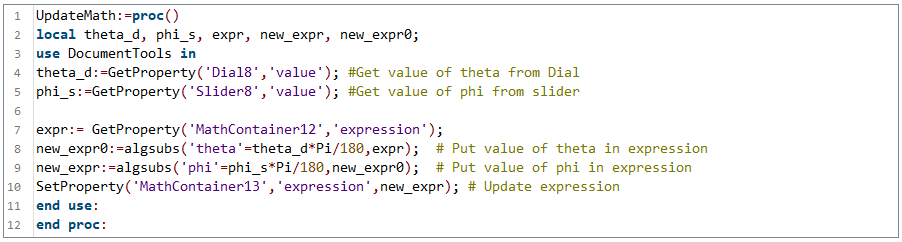```UpdateMath:=proc()
local theta_d, phi_s, expr, new_expr, new_expr0;
use DocumentTools in
theta_d:=GetProperty('Dial8','value'); #Get value of theta from Dial
phi_s:=GetProperty('Slider8','value'); #Get value of phi from slider

expr:= GetProperty('MathContainer12','expression');
new_expr0:=algsubs('theta'=theta_d*Pi/180,expr);  # Put value of theta in expression
new_expr:=algsubs('phi'=phi_s*Pi/180,new_expr0);  # Put value of phi in expression
SetProperty('MathContainer13','expression',new_expr); # Update expression
end use:
end proc:```

 • Now change the code in the components to call the function using.

 • Since the code above is only defined there it will need to be run once (but only once) before moving the components. Instead of leaving it here you can add it to the Startup code by clickingor going to Edit > Startup code.  This code will run every time you open the Math App ensuring that it works right away.

 • The startup code isn't defined in this document to allow progression of these steps.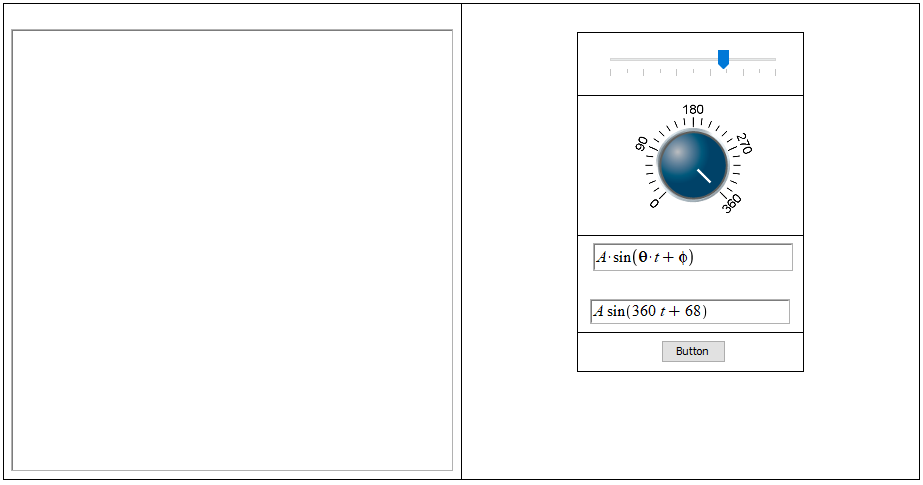Make the button initialize the app

 • Since the startup code isn't defined in this document we are going to move this function into the button.

UpdateMath:=proc()

```UpdateMath:=proc()
local theta_d, phi_s, expr, new_expr, new_expr0;
use DocumentTools in
theta_d:=GetProperty('Dial9','value'); #Get value of theta from Dial
phi_s:=GetProperty('Slider9','value'); #Get value of phi from slider

expr:= GetProperty('MathContainer14','expression');
new_expr0:=algsubs('theta'=theta_d*Pi/180,expr);  # Put value of theta in expression
new_expr:=algsubs('phi'=phi_s*Pi/180,new_expr0);  # Put value of phi in expression
SetProperty('MathContainer15','expression',new_expr); # Update expression
end use:
end proc:```
 • First click the button to rename it, you'll see theoption in the context panel on the right. Then add the code above to the button in the same way as the Slider an Dial (Right click and select Edit Click Code).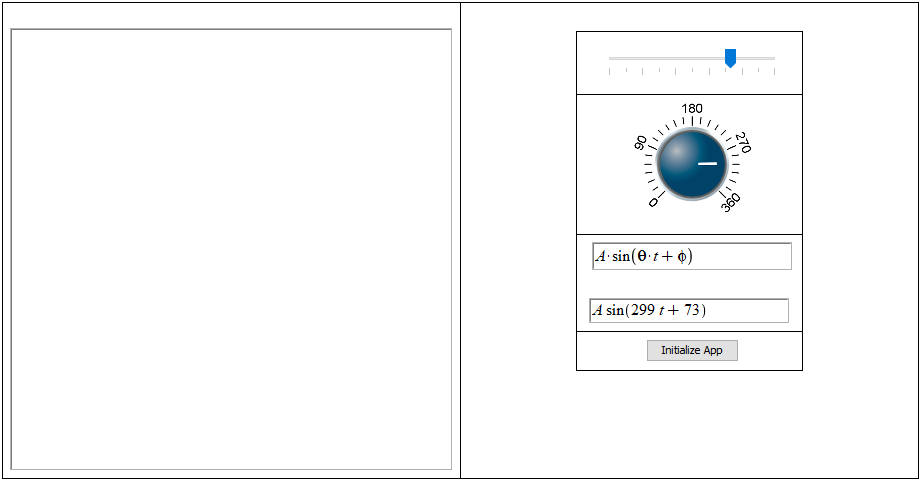Now it is easy to add new components

 • Now if we want to add new components we just have to change the one procedure.  Let's add a Volume Gauge to change the value of A.

 • Click in the cell containing the Dial, the context panel will show the option to Insert a row below the Dial.• Now drag a Volume Gauge into the new cell.

 • Click in the cell and choose the alignment (from the context panel) that looks best to you. In this case I chose center: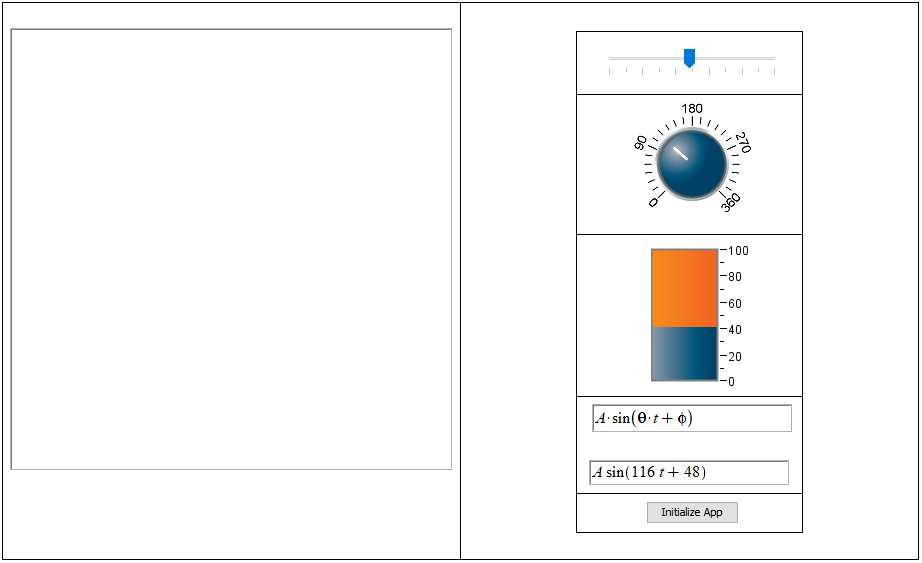Update the procedure code for the Gauge

 • Add two lines for the volume gauge to get the value and sub it into the expression
UpdateMath:=proc()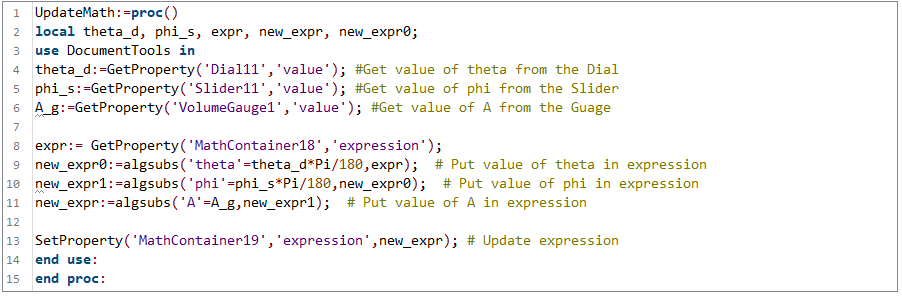```UpdateMath:=proc()
local theta_d, phi_s, expr, new_expr, new_expr0;
use DocumentTools in
theta_d:=GetProperty('Dial11','value'); #Get value of theta from the Dial
phi_s:=GetProperty('Slider11','value'); #Get value of phi from the Slider
A_g:=GetProperty('VolumeGauge1','value'); #Get value of A from the Guage

expr:= GetProperty('MathContainer18','expression');
new_expr0:=algsubs('theta'=theta_d*Pi/180,expr);  # Put value of theta in expression
new_expr1:=algsubs('phi'=phi_s*Pi/180,new_expr0);  # Put value of phi in expression
new_expr:=algsubs('A'=A_g,new_expr1);  # Put value of A in expression

SetProperty('MathContainer19','expression',new_expr); # Update expression
end use:
end proc:```
UpdateMath();

to the Gauge.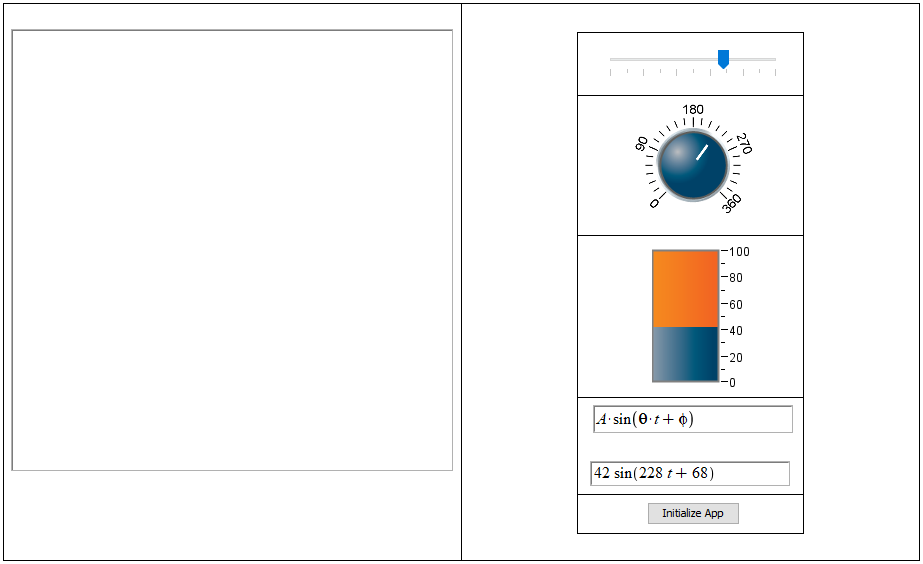Plot the changing expression

 • Make a procedure to get the value in the second Math Container and plot it

PlotMath:=proc()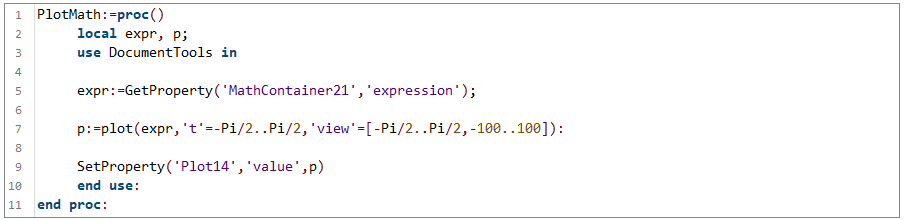```PlotMath:=proc()
local expr, p;
use DocumentTools in

expr:=GetProperty('MathContainer21','expression');

p:=plot(expr,'t'=-Pi/2..Pi/2,'view'=[-Pi/2..Pi/2,-100..100]):

SetProperty('Plot14','value',p)
end use:
end proc:```
 • Put this procedure in the Initialize button and the call to it in the components.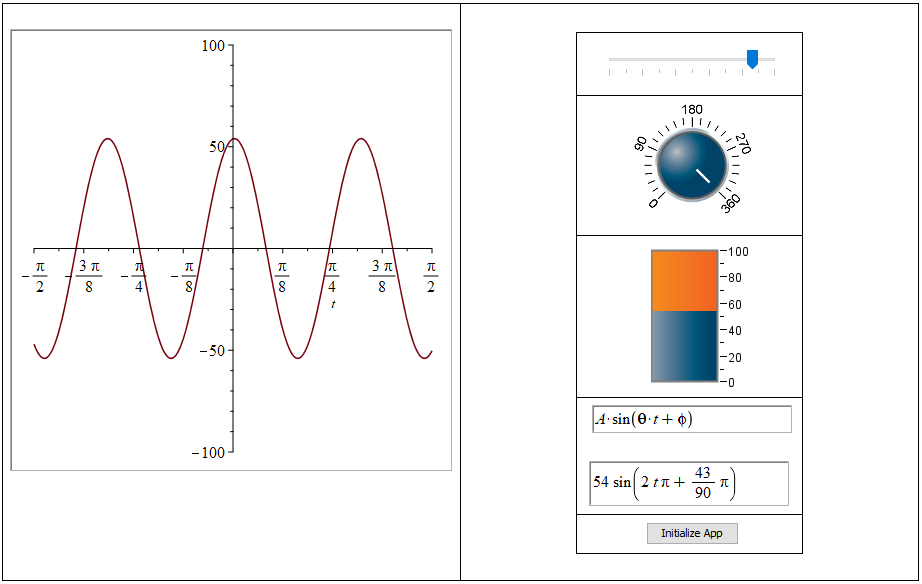Tidy up the app

 • Now that we have an interactive app let's tidy it up a bit.

 • The first thing I'd recommend in your own app is moving the code from the initialize button to startup code. In this document we choose to use the button instead to preserve earlier versions.

 • You can also remove the borders around the components by clicking in the table and selecting "Interior Borders" > "None" and "Exterior Borders" > "None" from the context panel.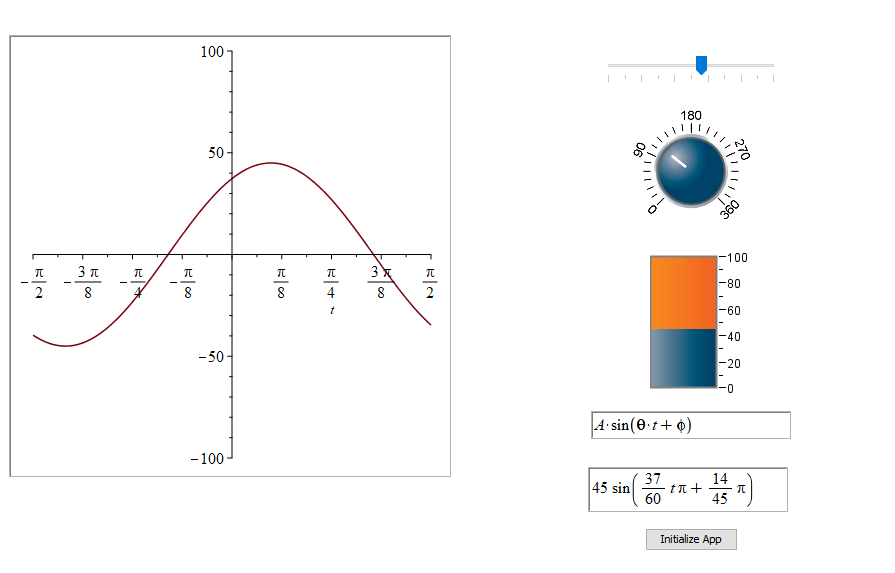Now you have a Math App

 • You can upload your Math App to the Maple Cloud to share with others by going to "File" > "Save to Cloud".

 • I'd recommend also including a description of what your app does. You can do this nicely using another table and Text mode.